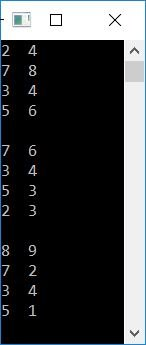# C Program for Three-Dimensional Array

In this article, you will learn how to program a three-dimensional array in C.

### How to Find the Size of a Three-Dimensional Array

To find the size of a three-dimensional array, say arr[i][j][k], use the following formula:

`size = i*j*k`

For example, if a given array is arr, then its size will be:

```size = 3*4*2
= 24```

That is, it can hold up to 24 elements.

## Three-Dimensional Array Program in C

An array of three dimensions, say arr, indicates a three two-dimensional array of size 4*2, which is the two dimensional array of four rows and two columns. Its initialization goes like this:

```arr = {
{ {1, 2}, {3, 4}, {5, 6}, {7, 8} },
{ {8, 7}, {6, 5}, {4, 3}, {2, 1} },
{ {1, 3}, {5, 7}, {9, 7}, {5, 3} }
};```

As you can see, there are three two-dimensional arrays with four rows and two columns each. Now let's move on to the program.

Three-dimensional (3D) arrays use three for loops in programming. Therefore, if you want to initialize the array dynamically or print its elements on output, you have to use three for loops. Like the program given below, which uses the same to print all the elements of a three-dimensional array on the output:

```#include<stdio.h>
#include<conio.h>
int main()
{
int i, j, k;
int arr = {
{ {2, 4}, {7, 8}, {3, 4}, {5, 6} },
{ {7, 6}, {3, 4}, {5, 3}, {2, 3} },
{ {8, 9}, {7, 2}, {3, 4}, {5, 1} }
};
for(i=0; i<3; i++)
{
for(j=0; j<4; j++)
{
for(k=0; k<2; k++)
printf("%d  ", arr[i][j][k]);
printf("\n");
}
printf("\n");
}
getch();
return 0;
}```

This program was built and runs in the Code::Blocks IDE. Here is its sample output:As you can see from the output given above, there are three two-dimensional arrays that get printed.

### Print 3D array elements and their indexes

To print all elements along with their index numbers. Here is the program:

```#include<stdio.h>
#include<conio.h>
int main()
{
int i, j, k;
int arr = {
{ {2, 4}, {7, 8}, {3, 4}, {5, 6} },
{ {7, 6}, {3, 4}, {5, 3}, {2, 3} },
{ {8, 9}, {7, 2}, {3, 4}, {5, 1} }
};
for(i=0; i<3; i++)
{
for(j=0; j<4; j++)
{
for(k=0; k<2; k++)
printf("arr[%d][%d][%d] = %d  ", i, j, k, arr[i][j][k]);
printf("\n");
}
printf("\n");
}
getch();
return 0;
}```

Here is its sample output:#### The same program in different languages

C Quiz

« Previous Program Next Program »